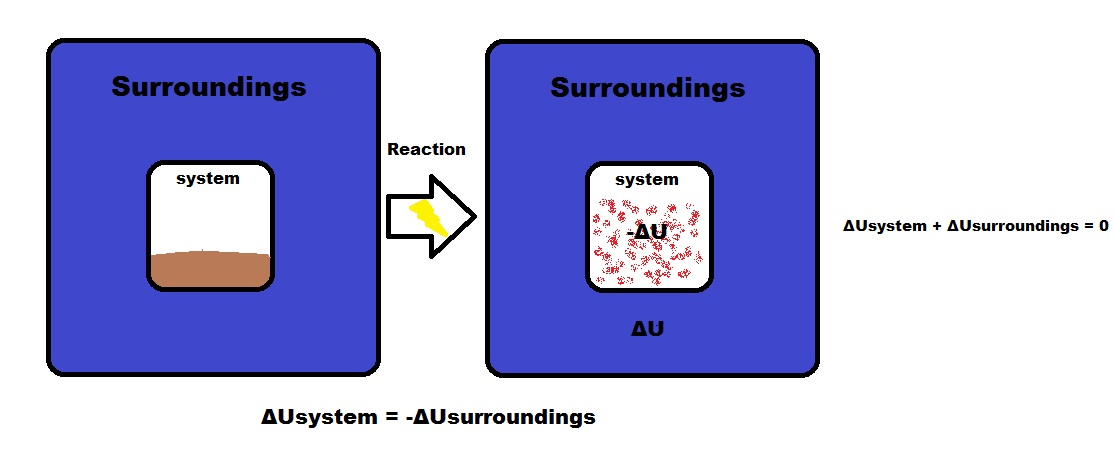# 11.1: Internal Energy

$$\newcommand{\vecs}{\overset { \rightharpoonup} {\mathbf{#1}} }$$ $$\newcommand{\vecd}{\overset{-\!-\!\rightharpoonup}{\vphantom{a}\smash {#1}}}$$$$\newcommand{\id}{\mathrm{id}}$$ $$\newcommand{\Span}{\mathrm{span}}$$ $$\newcommand{\kernel}{\mathrm{null}\,}$$ $$\newcommand{\range}{\mathrm{range}\,}$$ $$\newcommand{\RealPart}{\mathrm{Re}}$$ $$\newcommand{\ImaginaryPart}{\mathrm{Im}}$$ $$\newcommand{\Argument}{\mathrm{Arg}}$$ $$\newcommand{\norm}{\| #1 \|}$$ $$\newcommand{\inner}{\langle #1, #2 \rangle}$$ $$\newcommand{\Span}{\mathrm{span}}$$ $$\newcommand{\id}{\mathrm{id}}$$ $$\newcommand{\Span}{\mathrm{span}}$$ $$\newcommand{\kernel}{\mathrm{null}\,}$$ $$\newcommand{\range}{\mathrm{range}\,}$$ $$\newcommand{\RealPart}{\mathrm{Re}}$$ $$\newcommand{\ImaginaryPart}{\mathrm{Im}}$$ $$\newcommand{\Argument}{\mathrm{Arg}}$$ $$\newcommand{\norm}{\| #1 \|}$$ $$\newcommand{\inner}{\langle #1, #2 \rangle}$$ $$\newcommand{\Span}{\mathrm{span}}$$

The internal energy of a system is identified with the random, disordered motion of molecules; the total (internal) energy in a system includes potential and kinetic energy. This is contrast to external energy which is a function of the sample with respect to the outside environment (e.g. kinetic energy if the sample is moving or potential energy if the sample is at a height from the ground etc). The symbol for Internal Energy Change is $$ΔU$$.

Energy on a smaller scale

• Internal energy includes energy on a microscopic scale
• It is the sum of all the microscopic energies such as:
1. translational kinetic energy
2. vibrational and rotational kinetic energy
3. potential energy from intermolecular forces
Example

One gram of water at zero °Celsius compared with one gram of copper at zero °Celsius do NOT have the same internal energy because even though their kinetic energies are equal, water has a much higher potential energy causing its internal energy to be much greater than the copper's internal energy.

## Internal Energy Change Equations

The first law of thermodynamics states:

$dU=dq+dw$

where $$dq$$ is heat and $$dw$$ is work.

An isolated system cannot exchange heat or work with its surroundings making the change in internal energy equal to zero:

$dU_{\text {isolated system}} = 0$

Therefore, in an isolated system:

$dq=-dw$

### Energy is Conserved$dU_{\text {isolated system}} = dU_{\text {system}} + dU_{\text {surroundings}}$

$dU_{\text {system}}= -dU_{\text {surroundings}}$

### The signs of internal energy

• Energy entering the system is POSITIVE (+), meaning heat is absorbed, q>0. Work is thus done on the system, w>0
• Energy leaving the system is NEGATIVE (-), meaning heat is given off by the system, q<0 and work is done by the system, w<0

## Quick Notes

• A system contains ONLY Internal Energy
• A system does NOT contain energy in the form of heat or work
• Heat and work only exist during a change in the system; they are path functions
• Internal energy is a state function